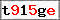This website uses cookies to improve user experience. By using our website you consent to all cookies in accordance with our Cookie Policy. X

### Basics of Stage3D part III: working with rotation [expert]

Continuing from where we left off this time around I want to write a bit about rotations. Anyone who started working with Flash's Stage3D without previous experience in 3D will sooner or later run into unforeseen results with appendRotation() function. To make things even less inviting for newcomers, looking for support on this topic on the Internet most routes lead to one answer "you will need complex numbers for that (quaternions to be exact). Hmm? Okay, quaternions might be really useful, but if you don't need 3D calculations to solve 2D rotations, it would be logical to assume that you also don't need 4D numbers to solve 3D problems, especially when being a newcomer to this topic.
Well, it doesn't matter right now so lets just move along, starting with the code from the previous post: Tutorial3D-2.zip, lets remove all those cubes and only leave one box, as that is all we need. By the way you could also create two additional variables: one for a Vector3D and one for Matrix3D - it will come in handy.
Generally rotation can be added in two ways: locally and globally, or in other words by either taking into the account the existing transformation or not. Which ever way we choose it is worth noting that existing transformation also includes any translation that might be applied to the matrix, which is why it is a good idea to make our lives easier and the rotation in separate Matrix3D object, only appending it to the existing object when needed. So lets start with the global transformation and creating a separate matrix (if you haven't done so already):
```private var rotMat:Matrix3D = new Matrix3D();
```
Next, we will modify the handling of keyboard arrow keys by including the rotation for rotMat:
```switch(key&0x0000ff) {
case 0x00000f: // forward
rotMat.appendRotation(SPEED, Vector3D.X_AXIS);
break;
case 0x0000f0: // backward
rotMat.appendRotation(-SPEED, Vector3D.X_AXIS);
break;
}
switch(key&0x00ff00) {
case 0x000f00: // left
rotMat.appendRotation(SPEED, Vector3D.Y_AXIS);
break;
case 0x00f000: // right
rotMat.appendRotation(-SPEED, Vector3D.Y_AXIS);
break;
}
```
Now only thing left to do is add that rotation to the box3D object, before it has its final transformation created in finalTransform:
```var boxMat:Matrix3D = box3D.getMatrix3D();
boxMat.identity();
boxMat.append(rotMat);
```
Which should result in this:
To make this example a bit more interesting and also check if the rotation is correctly applied, that is without being affected by the translation, lets use the Vector3D I've mentioned earlier. Like this:
```private var posVec:Vector3D = new Vector3D();
```
Working with vectors should not bring any troubles at this stage, so I will only mention we will have to append it in proper place - right before the boxMat.append(rotMat); insert this line:
```boxMat.appendTranslation(posVec.x, posVec.y, posVec.z);
```
In turn getting this:
So the global transformation isn't really that complicated, but there is still the matter of local rotation. Fortunately it is quite trivial and comes down to only finding the right axis:
```switch(key&0x0000ff) {
case 0x00000f: // forward
rotMat.appendRotation(SPEED, rotMat.transformVector(Vector3D.X_AXIS));
posVec.y += SPEED/50;
break;
case 0x0000f0: // backward
rotMat.appendRotation(-SPEED, rotMat.transformVector(Vector3D.X_AXIS));
posVec.y -= SPEED/50;
break;
}
switch(key&0x00ff00) {
case 0x000f00: // left
rotMat.appendRotation(SPEED, rotMat.transformVector(Vector3D.Y_AXIS));
posVec.x -= SPEED/50;
break;
case 0x00f000: // right
rotMat.appendRotation(-SPEED, rotMat.transformVector(Vector3D.Y_AXIS));
posVec.x += SPEED/50;
break;
}
```
The X_AXIS vector will be transformed the object's local system, which will give us the exact location of the X axis we will use for the rotation. If done right at least one edge (depending on the direction of the rotation) will stay in same place (minus the translation):
Source code: Tutorial3D_rot.zip

Name:
Comment:
Confirm the image code: